# Average height

There are twice as many girls in the class as there are boys. The average height of girls is 177 cm, boys 186 cm. What is the average height of students in this class?

a =  180 cm

### Step-by-step explanation: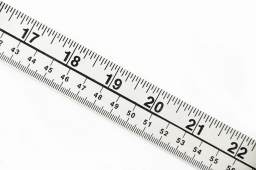Did you find an error or inaccuracy? Feel free to write us. Thank you!Tips to related online calculators
Looking for help with calculating arithmetic mean?
Looking for a statistical calculator?
Do you want to convert length units?

#### You need to know the following knowledge to solve this word math problem:

We encourage you to watch this tutorial video on this math problem:

## Related math problems and questions:

• Average height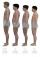In a class are 34 students. The average height of the students is 165 cm. What will be the average height of students in the classroom when two pupils, tall 176 cm and 170 cm, moved from this school/class? The average height in meters of all the students
• Average heightThe average height of all pupils is 162 cm. The class teacher's height is 178 cm. The average height of all (teacher and all pupils) is 163 cm. Calculate the number of pupils in the class.
• The average heightThe average height of six friends is 181 cm. Peter came among them, which reduced the average height by 2 cm. Calculate Peter's height.
• ClassroomThere are eighty more girls in the class than boys. Boys are 40 percent and girls are 60 percent. How many are boys and how many girls?
• Final examThere are 5 learners in a class that has written a final exam Aleta scored 55% vera scored 36% and Sibusiso scored 88% if Thoko scored 71% and the class average was 63%. What was Davids score as a percentage?
• Prospering studentsThere are girls and 30 boys in the class. Boys prospering 28, girls all. How many girls are in the class and how many boys are there, if all the prospering students are 95%?
• Statistics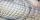The sum of all deviations from the arithmetic mean of the numerical sequence 4, 6, 51, 77, 90, 93, 95, 109, 113, 117 is:
• Certificate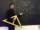There is 31 students in a class. From mathematics was'nt worse mark than 2. Average mark in mathematics was 2. How many students have mark 1 and how many mark 2?
• Marks at schoolThere are 30 students in the class. Five students in the class had a mark three triple at the end of the math certificate, and the other students had a mark of one or two. The average mark in all students' mathematics in the class at the end of the year w
• TrayWhat height reach water level in the tray shaped a cuboid, if it is 420 liters of water and bottom dimensions are 120 cm and 70 cm.
• Annual incomeThe annual income (in thousands of \$) of fifteen families is 60, 80, 90, 96, 120, 150, 200, 360, 480, 520, 1060, 1200, 1450, 2500, 7200. Calculate the harmonic and geometric mean.
• SchoolsThree schools are attended by 678 pupils. To the first attend 21 students more and to the third 108 fewer students than to second school. How many students attend the schools?
• Train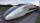A passenger train traveled for 2 hours 74 km. 3.1 hours after its departure started fast train and caught it on 186 km. How many km/h is different its average speeds?
• Boys and girlsThere are eight boys and nine girls in the class. There were six children on the trip from this class. What is the probability that left a) only boys b) just two boys
• Jane classWhen asked how many students are in class, Jane said, if we increase the number of students in our class by hundred % and then add half the number of students, we get 100. How many students are in Jane's class?
• A isoscelesA isosceles triangle has an area of 168 cm2 and it's added height and base is 370 cm. What are the measurements of it's height and base?
• Test scoresJo's test scores on the first four 100 point exams are as follows: 96,90,76, and 88. If all exams are worth the same percent, what is the minimum test score necessary on his last exam to earn an A grade in the class (90% or better)?